Open In App
Related Articles
• CBSE Class 9 Maths Revision Notes
• CBSE Class 9 Maths Formulas
• NCERT Solutions for Class 9 Maths
• RD Sharma Class 9 Solutions

# Algebraic Identities

Algebraic Identities are some of the fundamental concepts of algebra which lay the foundation of the complete stream of mathematics. They are very useful for performing large complex calculations with ease, for example, if we have to multiply 198 and 211 and need our answers in such a way that demands less computing power, it can be written as (200-2)(200+11), which now can easily be simplified using one the identities. Identities are used in algorithms to make them efficient and less computing power-intensive as well by the students to help them simplify complex calculations into simple steps.

## What are Algebraic Identities?

An identity is a mathematical equation that remains true regardless of the values assigned to its variables. They are useful in simplifying or rearranging algebraic expressions because the two sides of identity are interchangeable, they can be swapped with one another at any point. For example, x2=4, 2x-7=4, x3+2x2+5=7x, etc. are only satisfied by some values, so these are not examples of identities. On the other hand, (x+2)2=x2+4x+4, satisfy all the real value for x, so it is an example of identity.

## List of Algebraic Identities

There are a lot of identities since we can change the expression used in identity a little bit and call it another identity.

For example, for (a – b)2 = a2 + b2 -2ab

Now a can be changed to ax, and it will form a new identity, i.e., ax + b)(ax – b) = ax2 – b2

There are some identities considered Standard identities by the mathematics community so that there can be some common ground for all the maths students around the globe.

Some Standard identities are as follows,

There are two more identities, which can be derived from a cube of sum and cube of difference identities, as follows:

As we know, (a+b)3 = a3+b3+3ab(a+b)

⇒ (a+b)3 – 3ab(a+b)= a3+b3

⇒ (a+b)((a+b)2 – 3ab)= a3+b3

Using, (a + b)2 = a2 + b2 + 2ab, in above equation

⇒ (a+b)(a2+ b2+2ab- 3ab)= a3+b3

⇒ a3+b3= (a+b)(a2+ b2– ab)

Similarly, Using (a-b)3 = a3-b3-3ab(a-b),

As we know, (a-b)3 = a3-b3-3ab(a-b)

⇒ (a-b)3 +3ab(a-b)= a3-b3

⇒ (a-b)((a-b)2 + 3ab)= a3-b3

Using, (a – b)2 = a2 + b2 – 2ab, in above equation

⇒ (a-b)(a2+ b2-2ab+ 3ab)= a3-b3

⇒ a3-b3= (a-b)(a2+ b2+ ab)

## Proof of Algebraic Identities

Algebraic Identities can be proven either using algebraic methods or using visual methods, proofs for standard identities are as follows:

### Proof of (a+b)2=a2+2ab+b2

Visual Proof

For proof of (a+b)2= a2 + 2ab + b2 identity, let’s take a square of side a+b and divide it like the following diagram.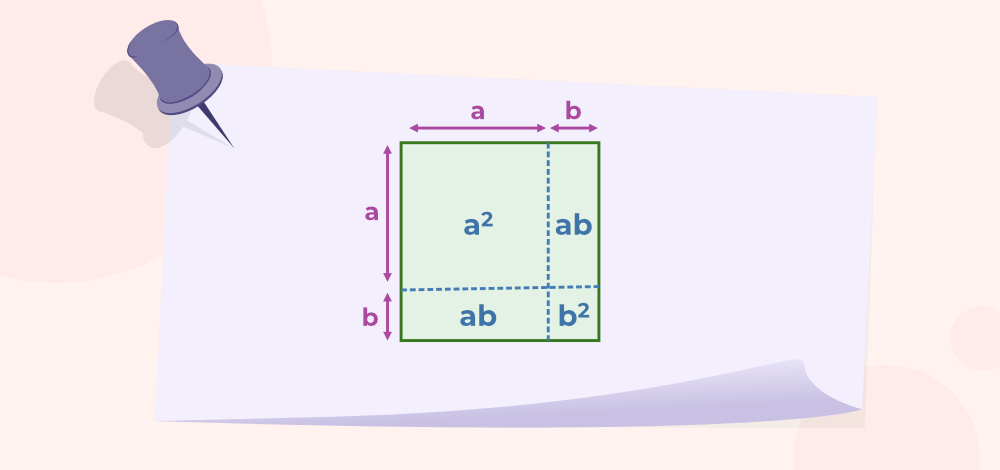Now, as the area of any geometric object doesn’t change if it is divided into any number of finite objects. Here, area before the division of square is (a+b)2, and after division, a2 + ab + ab + b2 i.e., a2 + 2ab + b2

Hence, proved the identity,  (a+b)2 = a2 + 2ab + b2

Algebraic Proof

(a + b)2 = (a + b)(a + b)    [Using law of exponent]

⇒ (a + b)2 =  a(a + b) + b(a + b)    [Using law of distribution]

⇒ (a + b)2 =  a2 + ab + ba + b2    [Using law of distribution]

Also, as multiplication is commutative, i.e., ab=ba

⇒ (a + b)2 =  a2 + ab + ab+ b2

⇒ (a + b)2 =  a2 + 2ab + b2

### Proof of (a-b)2=a2-2ab+b2

Visual Proof

For proof of (a-b)2=a2-2ab+b2 identity, let’s again consider a square but this time with side “a”.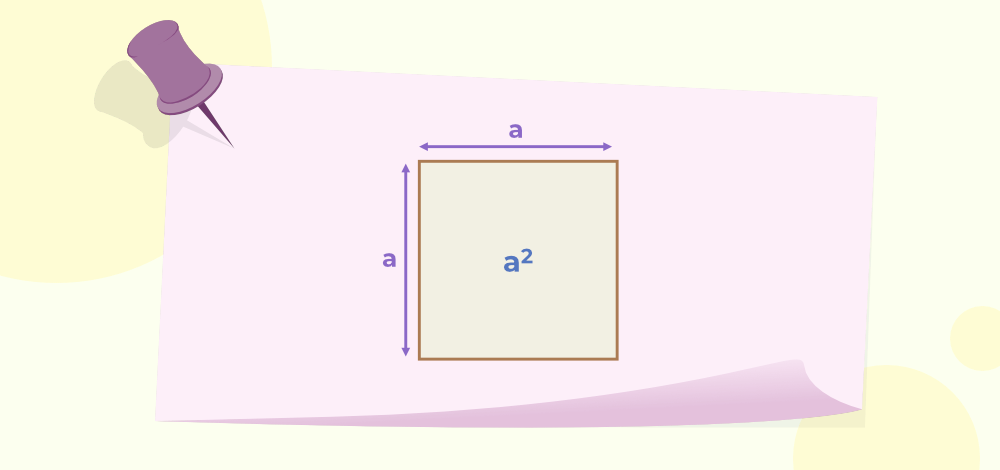Now, Let’s take a small segment “b” from its side and divide the square as follows: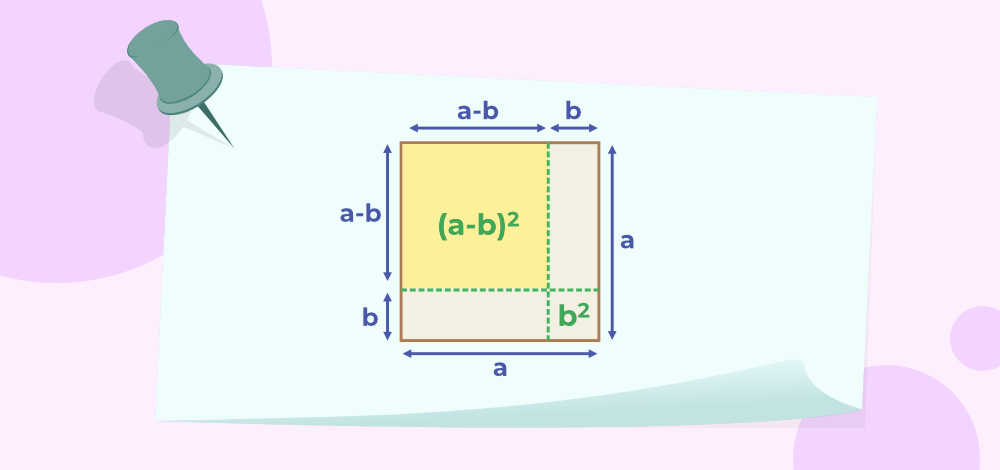To prove the identity, we have to calculate the area of the square with side (a-b) which is (a-b)2. Now, the initial area of the square is a2,  if both small strips with area ab are removed from area a2, the remaining area is a little less than the required area as we have subtracted the b2 part of the area twice, once in each strip. To compensate for this, by adding b2 back, we get out the required result. a2-2ab+b2, which is the required area of the square of side a-b.

Hence, proved the identity (a-b)2 = a2-2ab+b2.

Algebraic Proof

(a – b)2 = (a – b)(a – b)    [Using law of exponent]

⇒ (a – b)2 =  a(a – b) – b(a – b)    [Using law of distribution]

⇒ (a – b)2 =  a2 – ab – ba + b2    [Using law of distribution]

Also, as multiplication is commutative, i.e., ab=ba

⇒ (a – b)2 =  a2 – ab – ab+ b2

⇒ (a – b)2 =  a2 – 2ab + b2

### Proof of (a-b)(a+b)=a2-b2

Visual Proof

For proof of (a-b)(a+b)=a2-b2identity, let us consider a square of side a as follows:Now, Let’s take a small segment “b” from its side and divide the square as follows: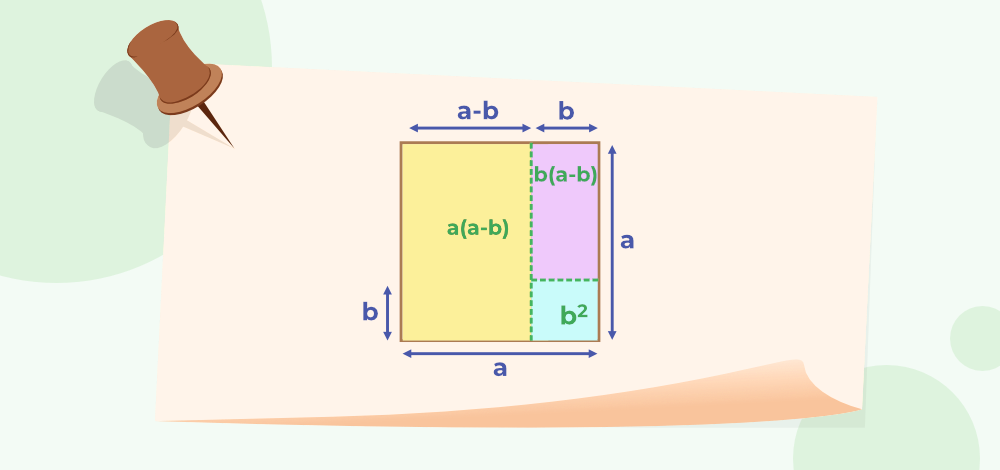To prove the required identity, we need to find the area of the square excluding the area of small square i.e., b2. The required area is the sum of both rectangles i.e., a(a-b)+b(a-b) = (a-b)(a+b).

Hence, proved the identity (a-b)(a+b)=a2-b2.

Algebraic Proof

(a-b)(a+b) = a(a + b) – b(a + b)    [Using law of distribution]

⇒ (a-b)(a+b) = a2 + ab – ba – b2    [Using law of distribution]

Also, as multiplication is commutative, i.e., ab=ba

⇒ (a-b)(a+b) = a2 + ab – ab – b2

⇒ (a-b)(a+b) = a2 – b2

### Proof of (x+a)(x+b)=x2+(a+b)x+ab

Visual Proof

Algebraic Proof

(x+a)(x+b)= x(x + b)+a(x + b)    [Using law of distribution]

⇒  (x+a)(x+b)= x2 + bx+ax + ab    [Using law of distribution]

⇒  (x+a)(x+b)= x2 + (a+b)x + ab

### Proof of (a+b+c)2=a2+b2+c2+2ab+2bc+2ca

Visual Proof

Let us consider a square with sides a+b+c and divide it as follows: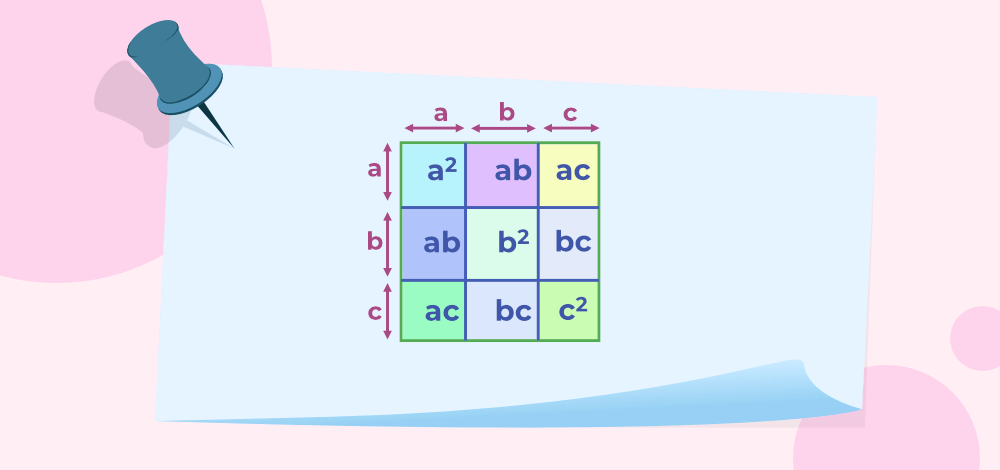Now, the initial area of the square is (a+b+c)2 using the formula for the area of the square and another way to find area is that adding all the small square areas. So, the sum of the area of all small squares is a2+ab+ac+ab+b2+bc+ac+bc +c2 which can be simplified to a2+b2+c2+2ab+2bc+2ca.

Hence, proved the identity (a+b+c)2=a2+b2+c2+2ab+2bc+2ca.

Algebraic Proof

To proof the above identity, let b+c = d

(a+b+c)2=(a+d)2

⇒  (a+b+c)2=a2+(b+c)2+2a(b+c)

⇒  (a+b+c)2=a2+b2+c2+2bc+2ab+2ac      [Using, (a+b)2=a2+2ab+b2]

⇒  (a+b+c)2=a2+b2+c2+2ab+2bc+2ac

### Proof of (a+b)3 = a3+b3+3ab(a+b)

Visual Proof

For proof of (a+b)3 = a3+b3+3ab(a+b) identity, let us consider a cube with side a+b as the following diagram,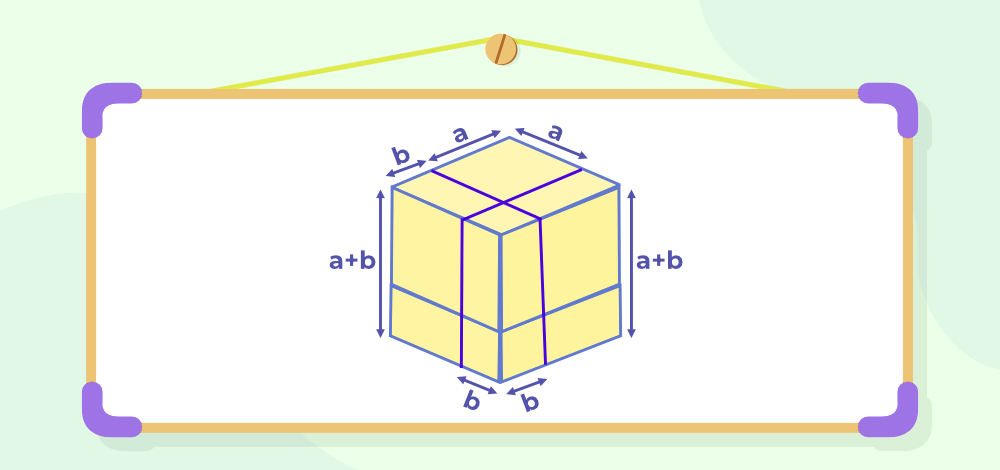Now, divide this cube into two cuboids to simplify and identify all the cuboids and cube which makes the volume of the original cube as follows: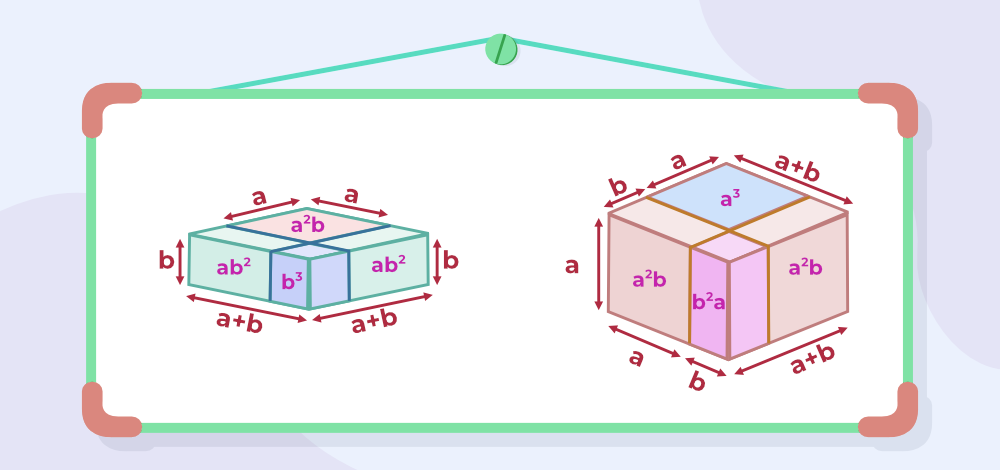Now, the initial volume of the Cube is (a+b)3, and the volume of small cubes also adds up to the same. The sum of the volume of small cubes is a3+a2b+b2a+ab2+a2b+ab2+ab2+b3 which can be simplified to a3+b3+3a2b+3ab2 or a3+b3+3ab(a+b).

Hence, proved the identity (a+b)3 = a3+b3+3ab(a+b).

Algebraic Proof

(a+b)3 = (a+b)(a+b)2

⇒(a+b)3 = (a+b)(a2+b2+2ab)   [Using, (a+b)2=a2+2ab+b2]

⇒(a+b)3 = a(a2+b2+2ab)+b(a2+b2+2ab)     [Using law of distribution]

⇒(a+b)3 = a3+ab2+2a2b+ba2+b3+2ab2       [Using law of distribution]

⇒(a+b)3 = a3+b3+3a2b+3ab2

⇒(a+b)3 = a3+b3+3ab(a+b)

### Proof of (a-b)3 = a3-b3-3ab(a-b)

Visual Proof

For proof of identity (a-b)3 = a3-b3-3ab(a-b), let us consider a cube with side a and a small segment of side a be b as follows: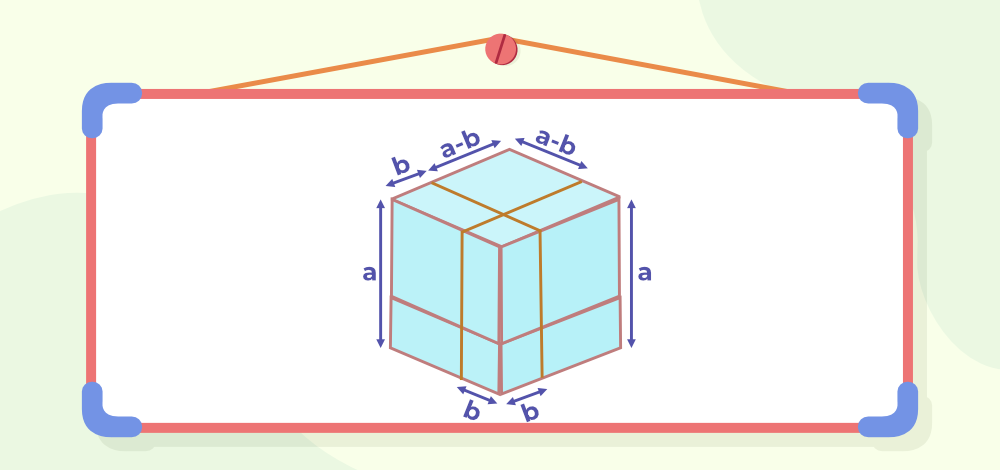Split the cubes into small chucks to easily calculate volume as follows: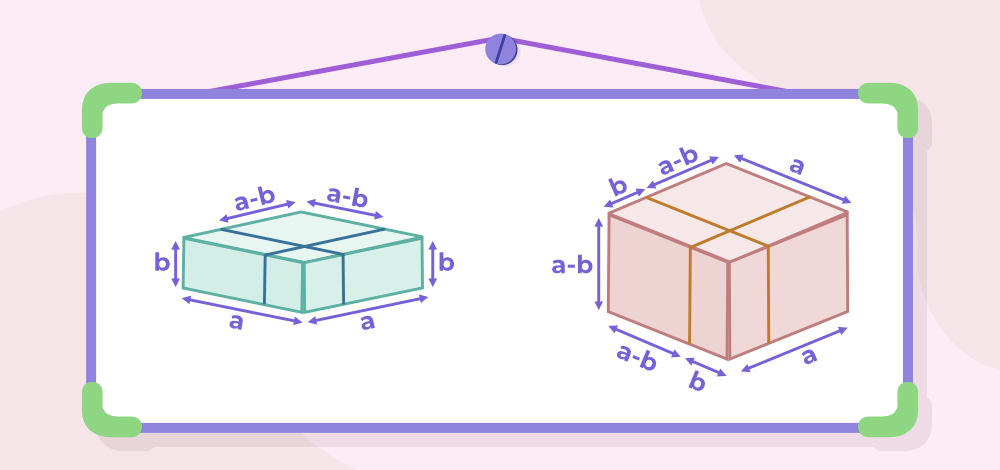and splitting these cuboids to more simplified cuboids and cubes as follows: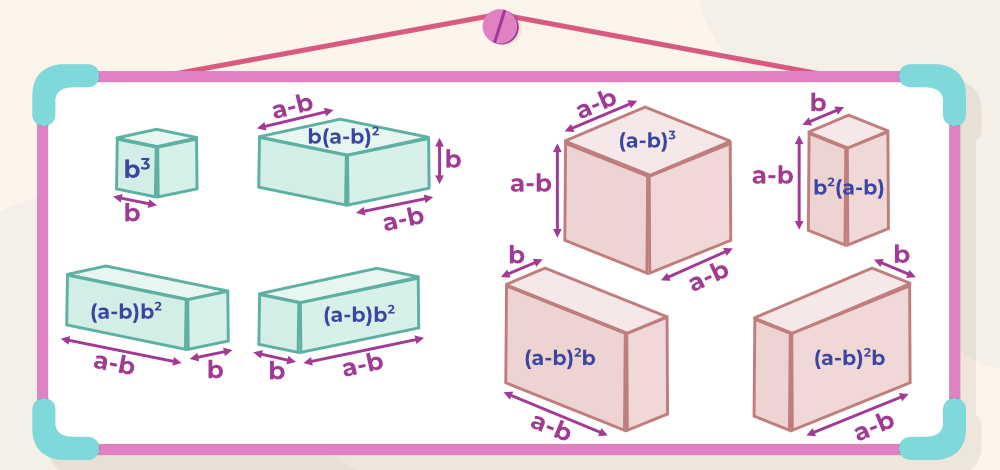Now, using the same volume remains constant before and after direction concepts.

a3=(a-b)3+b2(a-b)+(a-b)2b+(a-b)2b+b3+(a-b)2b+(a-b)b2+(a-b)b2

⇒ a3=(a-b)3+b(a-b)[b+a-b+a-b+a-b+b+b]+b3

⇒ a3=(a-b)3+b(a-b)[3a]+b3

⇒ a3=(a-b)3+3ab(a-b)+b3

Rearrenging this, we get (a-b)3 = a3-b3-3ab(a-b)

Hence, proved the identity (a-b)3 = a3-b3-3ab(a-b).

Algebraic Proof

(a-b)3 = (a-b)(a-b)2

⇒(a-b)3 = (a-b)(a2+b2-2ab)   [Using, (a-b)2=a2-2ab+b2]

⇒(a-b)3 = a(a2+b2-2ab)-b(a2+b2-2ab)     [Using law of distribution]

⇒(a-b)3 = a3+ab2-2a2b-ba2-b3+2ab2       [Using law of distribution]

⇒(a-b)3 = a3-b3-3a2b+3ab2

⇒(a-b)3 = a3-b3-3ab(a-b)

### Proof of a3 + b3 + c3 – 3abc = (a + b + c)(a2 + b2 + c2 – ab – bc – ca)

Algebraic Proof

Taking R.H.S of the identity,

(a+b+c)(a2+b2+c2–ab–bc–ca)=a(a2+b2+c2–ab–bc–ca)+b(a2+b2+c2–ab–bc–ca)+c(a2+b2+c2–ab–bc–ca)

⇒ (a+b+c)(a2+b2+c2–ab–bc–ca)=a3+ab2+ac2–a2b–abc–ca2+a2b+b3+bc2–ab2–b2c–abc+a2c+b2c+c3–abc–bc2–c2a

all the terms cancel out with their negative counterpart,

⇒ (a+b+c)(a2+b2+c2–ab–bc–ca)=a3+b3+c3–3abc

## Solved Examples of Algebraic Identities

Question 1: Simplify (2x-3y)2+(2x+3y)2.

Solution:

As we know, (a + b)2 =  a2 + 2ab + b2  and (a – b)2 =  a2 – 2ab + b2

adding both together, (a + b)2 +(a – b)2=  a2 + 2ab + b2 + a2 – 2ab + b2

⇒ (a + b)2 +(a – b)2=  2a2 + 2b2

here, a=2x and b=3y

(2x-3y)2+(2x+3y)2 = 2(2x)2+2(3y)2

⇒ (2x-3y)2+(2x+3y)2 = 2(4x2)+2(9y2)

⇒ (2x-3y)2+(2x+3y)2 = 8x2+18y2

Question 2: Expand (5x – 3y)2.

Solution:

This is similar to expanding (a – b)2 = a2 + b2 – 2ab.

where a = 5x and b = 3y,

So (5x – 3y)2 = (5x)2 + (3y)2 – 2(5x)(3y)

⇒ 5x – 3y)2 = 25x2 + 9y2 – 30xy

Question 3: Factorize (x6 – 1) using the identities mentioned above.

Solution:

(x6 – 1) can be written as (x3)2 – 12

This resembles the identity a2 – b2 = (a + b)(a – b).

where a = x3, and b = 1.

So, x6 – 1 = (x3)2 – 1 = (x3 + 1) (x3 – 1).

Question 4: If X+Y=7 and XY=12, then find the value of  X3 + Y3?

Solution:

As we know, (X+Y)3 = X3+Y3+3XY(X+Y)

Putting values of X+Y=7 and XY=12,

73=X3+Y3+3×12×7

⇒ 343 = X3+Y3+252

⇒ X3+Y3= 343 – 252

⇒ X3+Y3= 91

Thus, the value of X3 + Y3 is 91.

Question 5: Find the value of (x + 6)(x + 6) using algebraic identities when x = 3.

Solution:

(x+6)(x+6) can be re-written as (x + 6)2

It can be rewritten in this form, (a + b)2 = a2 + b2 + 2ab.

(x + 6)2 = x2 + 62 + 2(6x)

= x2 + 36 + 12x

Given, x = 3.

(x + 6)2 = 32 + 36 + 12(3)

= 9 + 36 + 36

= 81

Question 6: If a + b = 12 and ab = 35, what is a4 + b4

Solution:

a4 + b4 can be written as (a2)2 + (b2)2

And we know, (x + y)2 = x2 + y2 + 2xy

⇒ x2 + y2 = (x + y)2 -2xy

So, in this case, x = a2, y = b2 ;

a4 + b4 = (a2 + b2)2 – 2(a2)(b2

⇒ ((a+b)2 – 2ab)2 – 2(a2)(b2

⇒ ((12)2 – 2(35))2 – 2(35)2

⇒ 5475 – 2450

⇒ 3026

Question 7: The identity 4(z + 7)(2z – 1) = Az2 + Bz + C holds for all real values of z. What is A + B + C?

Solution:

Multiplying out the left side of the identity, we have

4(x + 7)(2x − 1) = 8x2 + 52x − 28.

This expression must be equal to the right-hand side of the identity, implying

8x2 + 52x – 28 = Ax2 + Bx + C,

So now comparing both sides of the equation.

A = 8, B = 52 ad C – 28.

A + B + C  = 8 + 52 – 28 = 32

Question 8: If a + b + c = 6, a2 + b2 + c2 = 14 and ab + bc + ca = 11, what is a3 + b3 + c3 – 3abc?

Solution:

We know this identity,

a3 + b3 + c3 – 3abc = (a + b + c)(a2 + b2 + c2 – ab – bc – ca)

Substituting the given values,

a3 + b3 + c3 -3abc = (6)(14 -11)

⇒ (6)(3) = 18